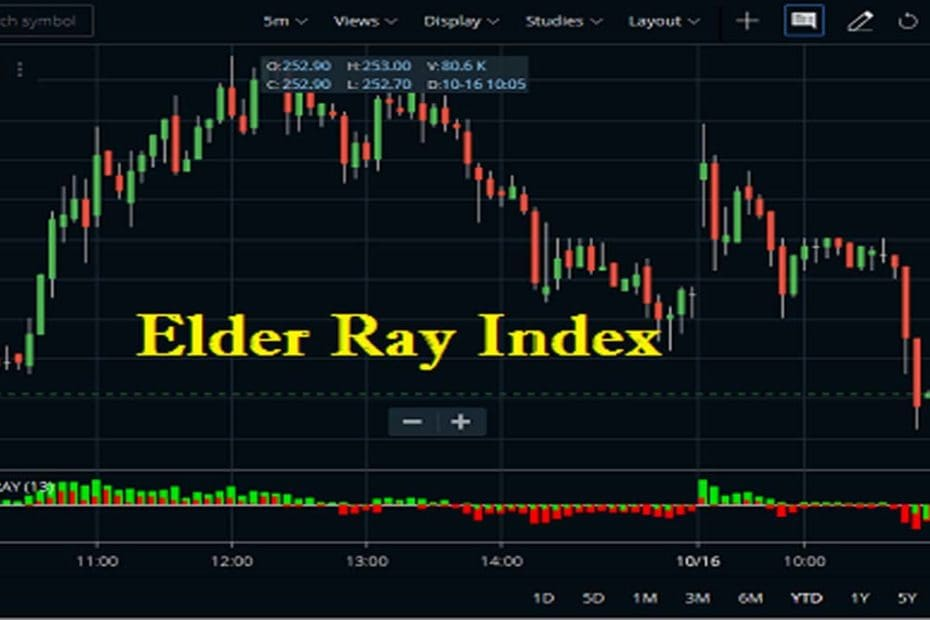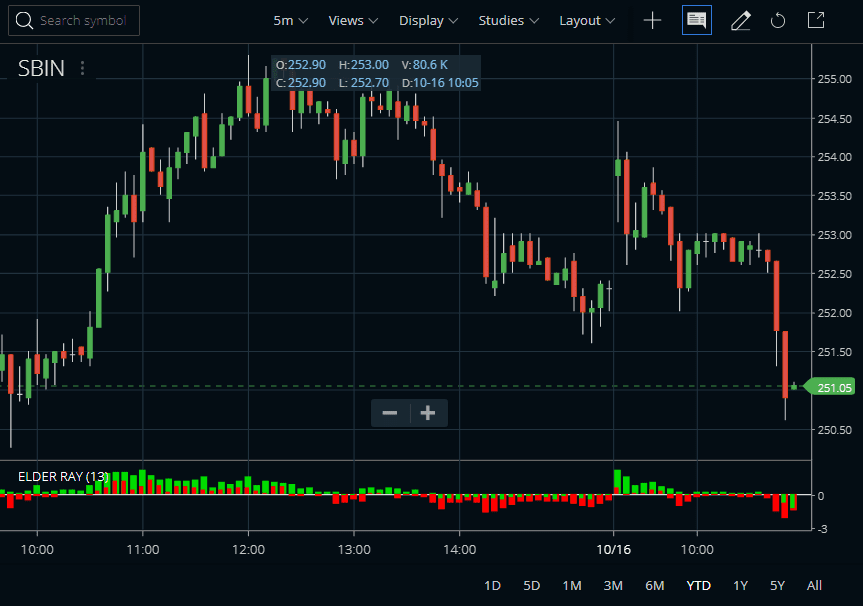Home » Blog » Trading » Technical Indicators » Elder Ray Index Indicator Strategy, Formula, Settings

# Elder Ray Index Indicator Strategy, Formula, Settings

• Technical IndicatorsOnce again, the Elder-Ray Index is an indicator developed by Alexander Elder. It measures the amount of buying and selling pressure in the market. The components of the Elder Ray Index indicator are “bull power” or “bear power”. The main part of this indicator is an exponential moving average, which is a trend-following indicator essential to the calculation.

Bull power of this indicator is a simple calculation. We can derive this by subtracting an exponential moving average of closing prices from a high price of any security. Similarly, we can calculate the Bear Power by subtracting the lowest price of any security from the exponential moving average.

The Elder Ray Index indicator is basically an oscillator. This indicator plots 2 lines as a histogram. One is the Bull Power and the other is the Bear Power. Bull Power indicates the buying strength in the market. While the Bear Power indicates the selling strength in the market.

## Elder Ray Index Indicator FAQ

How do you use the Elder Ray indicator?

In the Elder Ray Index indicator, Bull Power should remain positive under normal circumstances, and Bear Power should remain negative in normal circumstances. If Bull Power enters into negative territory, this implies that sellers have overcome buyers and control the market and the Bear Power indicator enters into positive territory and this indicator indicates that buyers have overcome sellers and control the market. A trader should not go long at times when the Bear Power indicator is positive and he/she should not go short at times when the Bull Power indicator is negative.

What is Bull Bear power?

The Elder Ray Index indicator consists of an exponential moving average. Bull Power is used to measure the potential for the price to increase above the moving average, and Bear Power is used to measure the potential for the price to decrease below the moving average. Long positions are taken when the Bear Power is below zero and there is a bullish divergence while short positions are assumed when the Bull Power is above zero and there is a bearish divergence.In this indicator, Bull Power is the result of subtracting the 13-period EMA from the high price of the day and Bear Power is the result of subtracting the 13-period EMA from the low price of the day.

## Elder Ray Index Indicator Formula

This indicator actually is derived from a moving average. Bull Power is a term to measure the potential for the price to increase above the moving average. On the other hand, Bear Power is a term to measure the potential for the price to decrease below the moving average.

In the Elder Ray Index indicator, the high price of any candle shows the maximum power of buyers and the low price of any candle shows the maximum power of sellers. In Zerodha Kite charts, it uses the EMA of 13-period by default. Similar parameters are also available on Upstox Pro.

See the State Bank of India chart image above, in order to present the average consensus of price value. In the chart, Bull Power shows whether buyers are capable of pushing prices above the average consensus of value. In this chart, the Bear Power shows whether sellers are capable of pushing prices below the average consensus of value.

## Elder Ray Index Indicator Strategy

Apart from the indicator let us attach a 13-period EMA on the same chart. We can use 13-period EMA’s slope in order to identify the direction of the major trend. This indicator can generate some most reliable buy signals. In this when there is a bullish divergence between the Bear Power indicator and the price. For example, the Bear Power forms higher lows, while the market forms lower lows. And in this indicator can also generate the most reliable sell signals, when there is a bearish divergence between the Bull Power indicator and the price. For example, Bull Power forms lower highs, while the market forms higher highs.

### The Trade Setup

As a perfect buy trade setup, look for the price above the 13-period exponential moving average. Now check if the EMA slope is up. Now check if the Bear Power is increasing or not. That means the Bear Power bar is higher than the previous bar. If all these criteria meet, open up a buy order.

Similarly, as a perfect short sell trade setup, look for the price below the 13-period exponential moving average. Now check if the EMA slope is down. Now check if the Bull Power is decreasing or not. That means the Bull Power bar is lower than the previous bar. If all these criteria meet, open up a short sell order. For the exit, you must keep a suitable stop loss and a well-calculated target.

This Elder Ray Index indicator is a part of the impulse system also developed by Alexander Elder. So to know more about the system you must read the book written by the developer. The best book in this subject will be Come Into My Trading Room: A Complete Guide to Trading (Wiley Trading).

## Conclusion

The Elder Ray Index indicator is an oscillator. This indicator calculates the price strength with the help of an exponential moving average. The 2 components of the indicator are Bull Power and Bear Power. The Bull Power measures the buying strength, while the Bear Power measures the selling strength. Combining this indicator with some other indicators can create a powerful trading system.